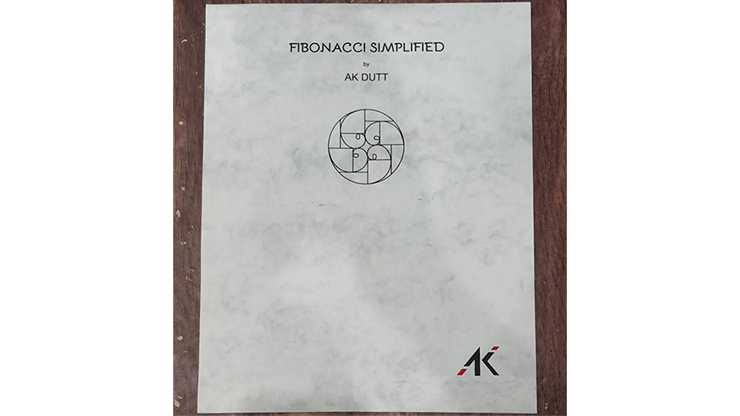## Black Label

At the Table Experience
The Vault

## Books & Magazines

Supreme Books (Limited)
FIBONACCI SIMPLIFIED by AK Dutt
FIBONACCI )  Trick
 R: \$9.95
Difficulty:BeginnerDescription:

A stupendous mathematical calculation by magic!

You first explain the idea behind Fibonacci calculations and provide some examples. Then, you astound your audience with your dynamic mathematical abilities!

This is the new SUPER FAST method of Fibonacci calculation.

For those "in the know," you need not wait until the 7th row is filled to reveal/predict the total of 10 Fibonacci numbers.

Now, you can reveal the total of 10 Fibonacci numbers soon after the 2nd row is filled.

In other words, you can perform this in less than half the time of the old method.

Two methods are explained in detail:
• Simple Method (with gimmick)